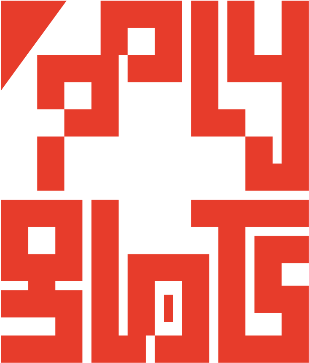polygl0ts

EPFL's CTF teamSquare CTF 2018 - C2: flipping bits

Description

Disabling C2 requires cracking a RSA message. You have two ciphertexts. The public key is (e1, n).

Fortunately (this time), space rabiation caused some bit flibs and the second ciphertext was encrypted with a faulty public key (e2, n). Can you recover the plaintexts?

ct1 = 13981765388145083997703333682243956434148306954774120760845671024723583618341148528952063316653588928138430524040717841543528568326674293677228449651281422762216853098529425814740156575513620513245005576508982103360592761380293006244528169193632346512170599896471850340765607466109228426538780591853882736654
ct2 = 79459949016924442856959059325390894723232586275925931898929445938338123216278271333902062872565058205136627757713051954083968874644581902371182266588247653857616029881453100387797111559677392017415298580136496204898016797180386402171968931958365160589774450964944023720256848731202333789801071962338635072065
e1 = 13
e2 =  15
modulus =  103109065902334620226101162008793963504256027939117020091876799039690801944735604259018655534860183205031069083254290258577291605287053538752280231959857465853228851714786887294961873006234153079187216285516823832102424110934062954272346111907571393964363630079343598511602013316604641904852018969178919051627

You have two captured ciphertexts. The public key is (e1, n). But,
due to a transient bit flip, the second ciphertext was encrypted with a faulty
public key: (e2, n). Recover the plaintexts.

(The algorithm is RSA.)

Solution

We are provided with two encodings of the same plaintext, using the same modulo but different public keys.

Further, we have gcd(e1, e2) = gcd(15, 13) = 1. This means that some values u and v, so that u*e1 + v*e2 = 1 holds, exist. We can easily find u and v thanks to the extended euclidian algorithm.

Now we notice that ct1 = M^e1 (mod n) and ct2 = M^e2 (mod n). We compute:

ct1^u * ct2^v = M^(u*e1) * M^(v*e2) = M^(u*e1 + v*e2) = M

Once we have M, all what remains to do is to take its binary representation and decode it as an ASCII string.

flag-54d3db5c1efcd7afa579c37bcb560ae0

Code

You can find the sage script we used to get the flag here.